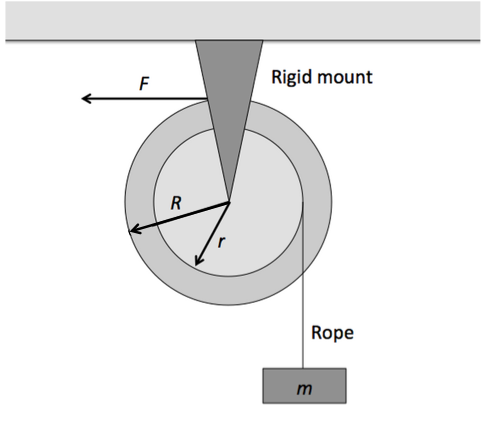Classical Mechanics

# Torque - Dynamical Behavior

The position of a $$3 \text{ kg}$$ particle moving on the $$xy$$-plane at time $$t$$ (in seconds) is given by the equation $$\vec{r} = \left( 2 t^2 \hat{i} - (4 t + 4 t^2) \hat{j} \right) \text{ m} .$$ Considering the origin $$O$$ as the pivot, what is the magnitude of the torque on the particle at $$t= 6 \text{ s}?$$A fixed pulley-like device is used to lift a box of mass $$m=13\text{ kg},$$ as shown in figure above. The outer radius of the device is $$R=7 \text{ m},$$ and the radius of the hub is $$r= 2 \text{ m}.$$ When a constant horizontal force $$F = 130 \text{ N}$$ is applied to a rope wrapped around the outer rim of the device, the box hanging from a rope wrapped around the hub acquires an upward acceleration of magnitude $$2 \text{ m/s}^2.$$ Then what is the rotational inertia of the device about its axis of rotation?

The gravitational acceleration is $$g= 10 \text{ m/s}^2.$$

A particle moves clockwise in a circle around the origin on the $$xy$$-plane. If its angular momentum as a function of time $$t$$ (in seconds) is $$5 t^2 \text{ kg} \cdot \text{m}^2\text{/s},$$ what is the magnitude of the torque on the particle at $$t = 4 \text{ s}?$$

A $$6 \text{ kg}$$ particle that moves on the $$xy$$-plane with velocity $$\vec{v} = \left( 6 \hat{i} + 2 \hat{j} \right) \text{ m/s}$$ is at $$x= 9 \text{ m}$$ and $$y= 12 \text{ m}.$$ It is being pulled by a $$6 \text{ N}$$ force in the $$-x$$ direction. Considering the origin as the pivot, what is the torque of the force?

A cubic dice is sliding on a frictionless table. The dice is a cube with edges of length 1 cm and a mass of 30 g. A kid reaches down and gives a horizontal flick to the dice, causing it to change which face is up. What is the minimum impulse in g~cm/s the kid must give to the dice in order to change the face?

Details and assumptions

• The acceleration of gravity is $$-9.8~m/s^2$$.
• You can model the dice as a perfect cube of uniform density.
• The dice never leave the table
×

Problem Loading...

Note Loading...

Set Loading...